# RD Sharma Solutions for Class 6 Maths Chapter 10: Basic Geometrical Concepts

Mathematics is one among the major scoring subjects which requires great practice. The students can study the content of other subjects easily but solving problems in Mathematics requires conceptual knowledge. The geometrical concepts explained in RD Sharma Solutions help in understanding the formation of various shapes using planes, points and lines.

The major applications of geometrical concepts are area of a land, astronomy, gap between two things etc. The solutions are designed by teachers at BYJU’S to make the concepts easier for the students while learning. RD Sharma Solutions for Class 6 Chapter 10 Basic Geometrical Concepts PDF can be downloaded here by the students.

## RD Sharma Solutions for Class 6 Maths Chapter 10: Basic Geometrical Concepts Download PDF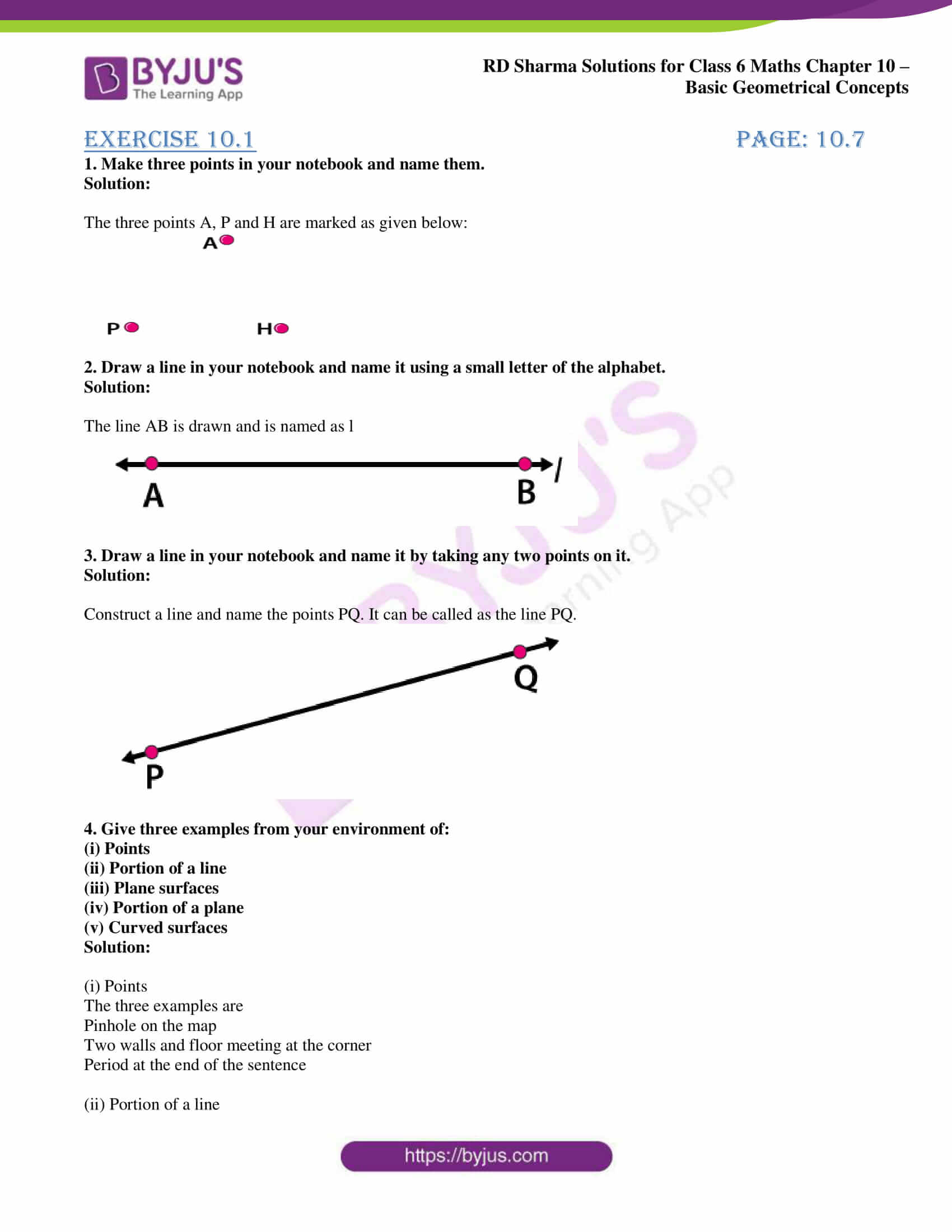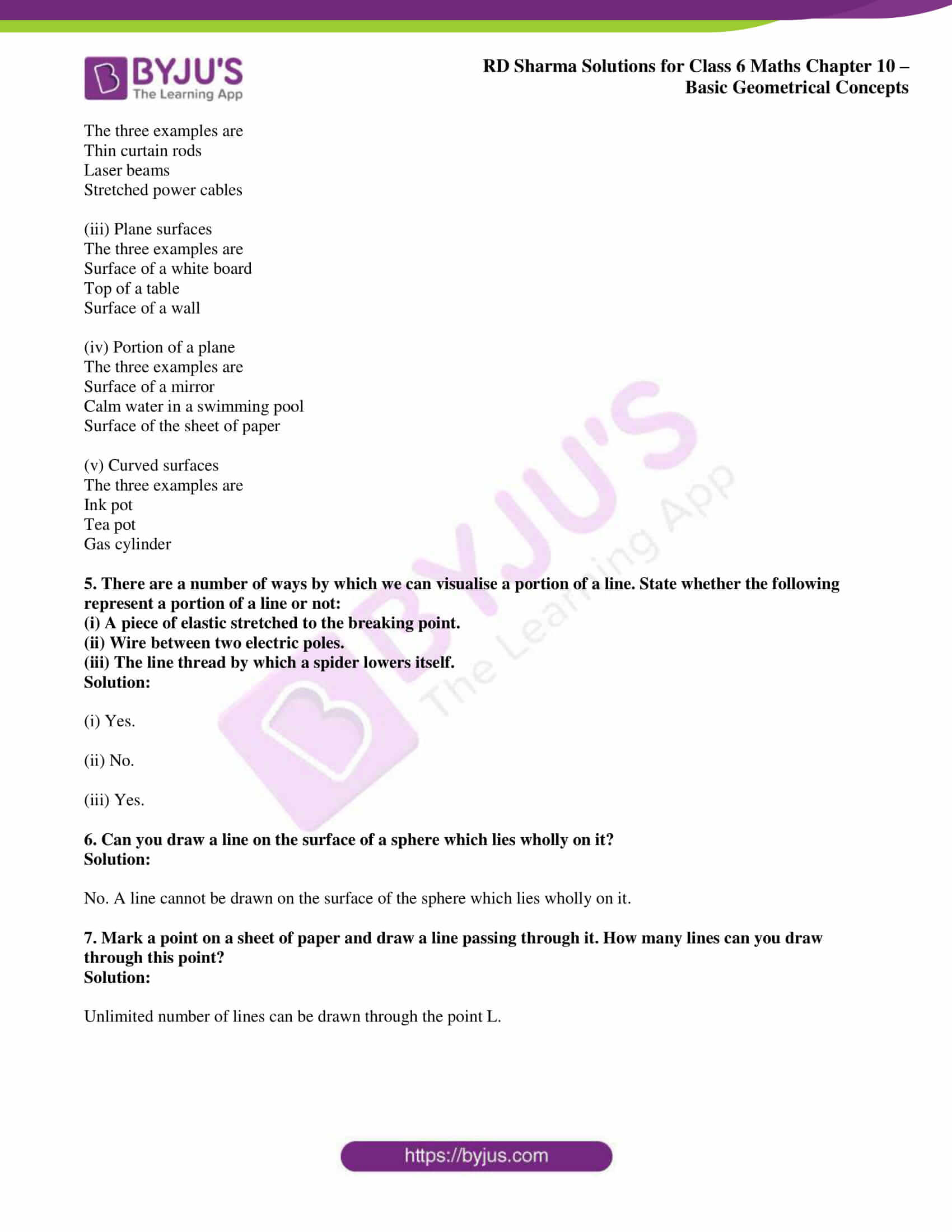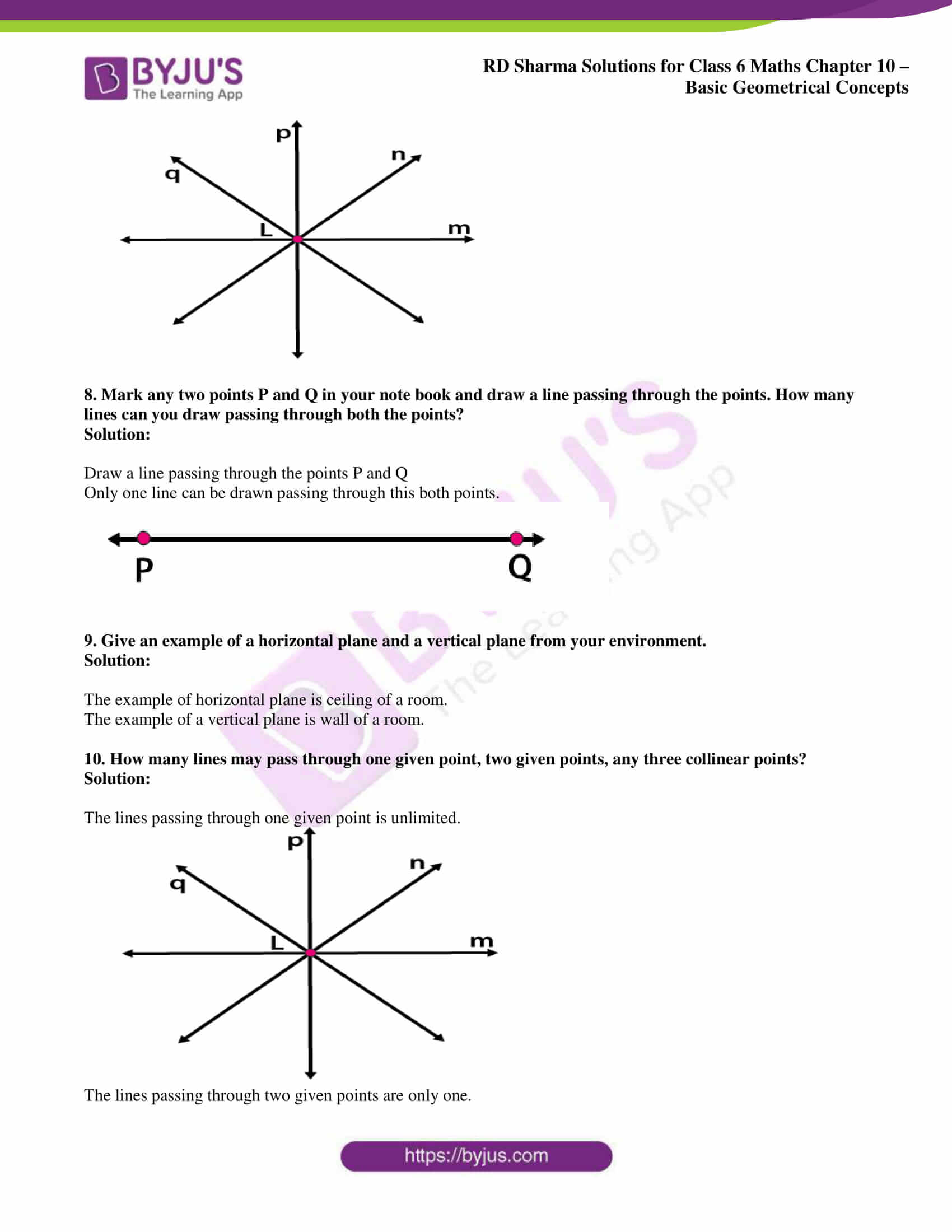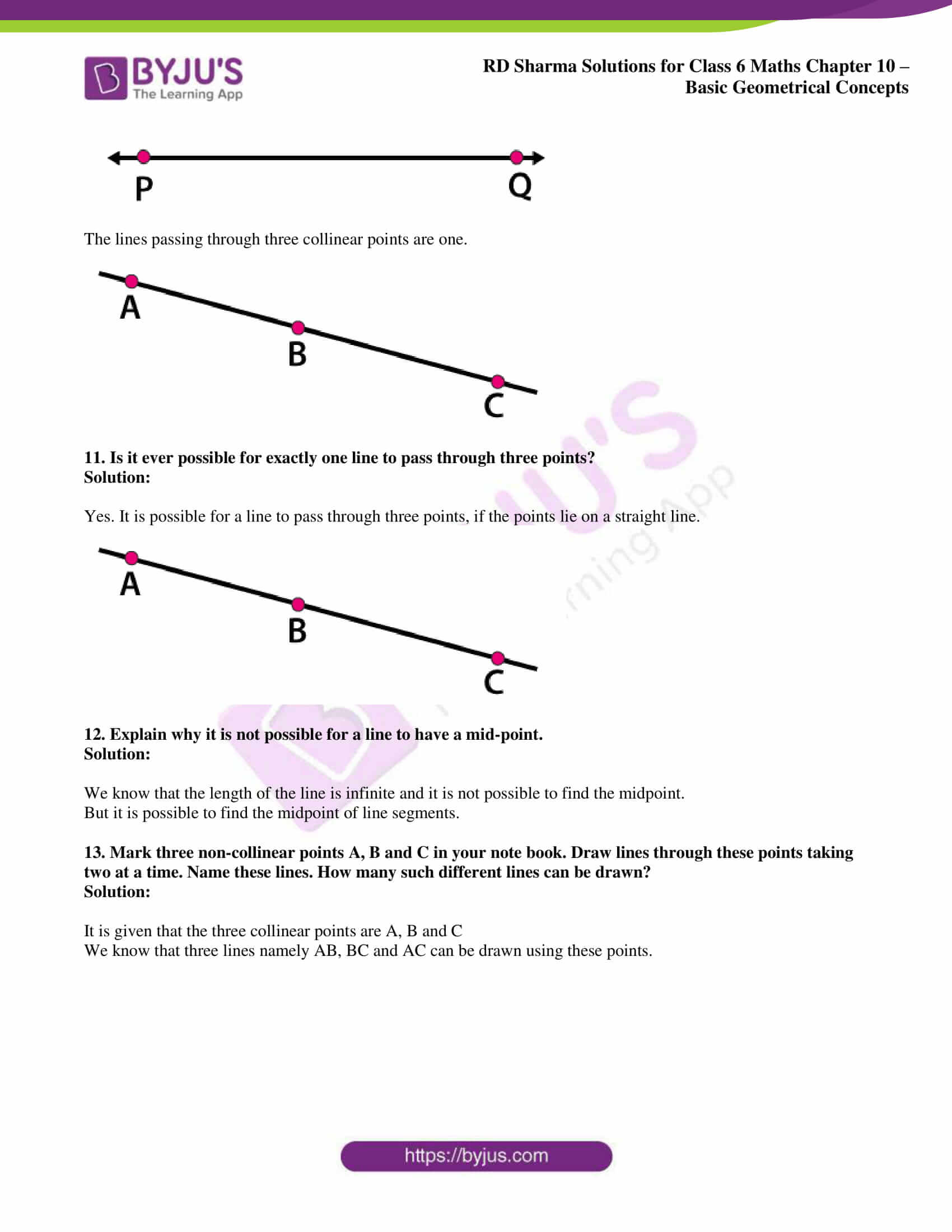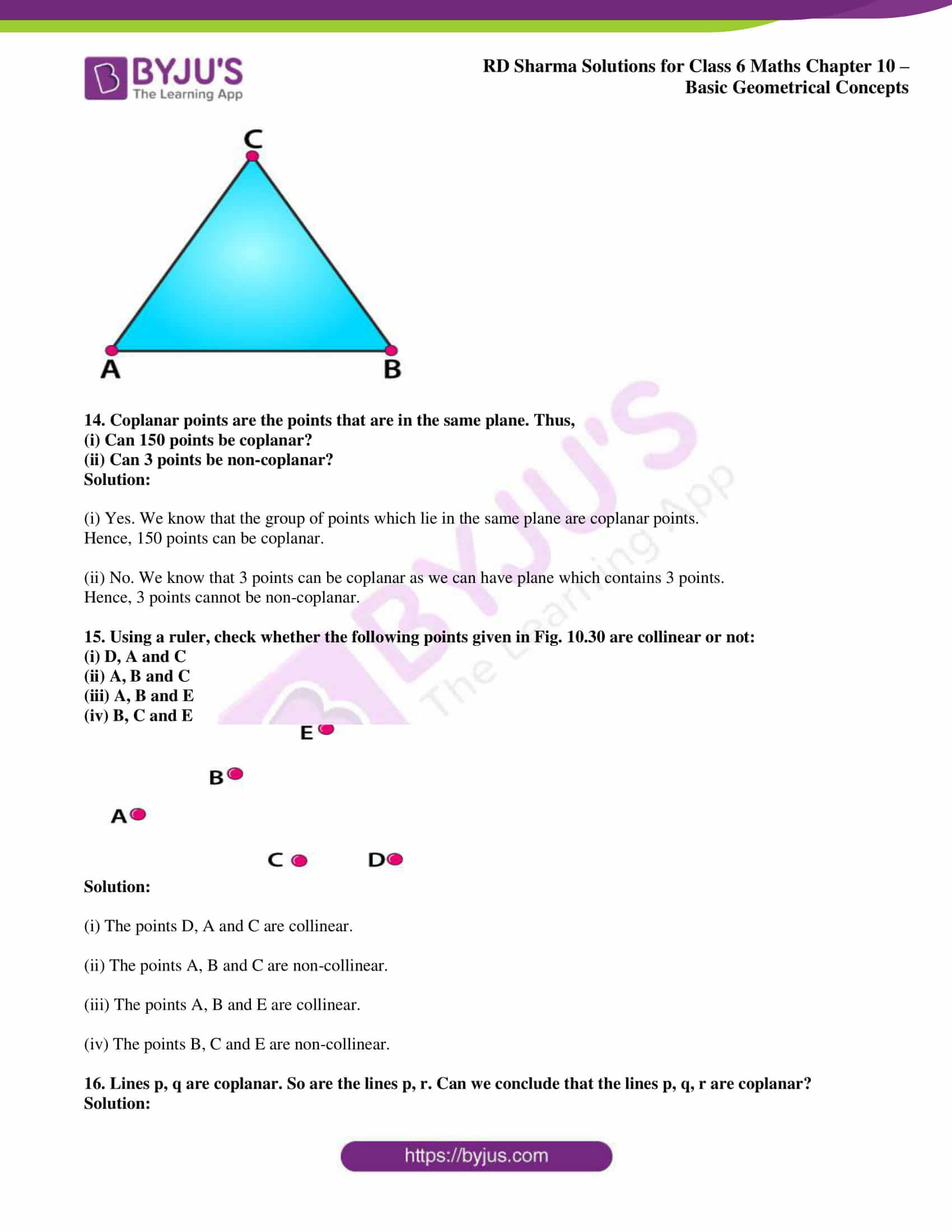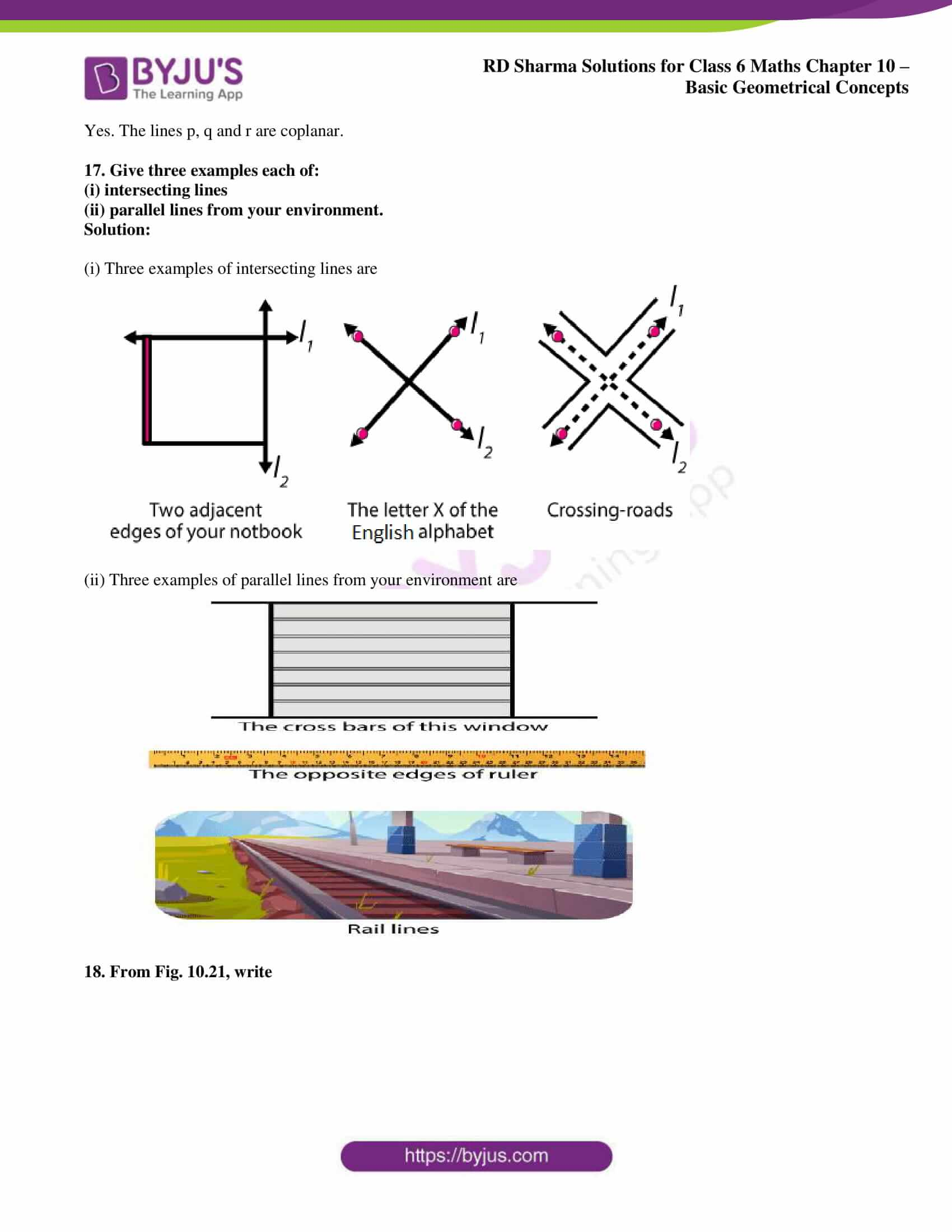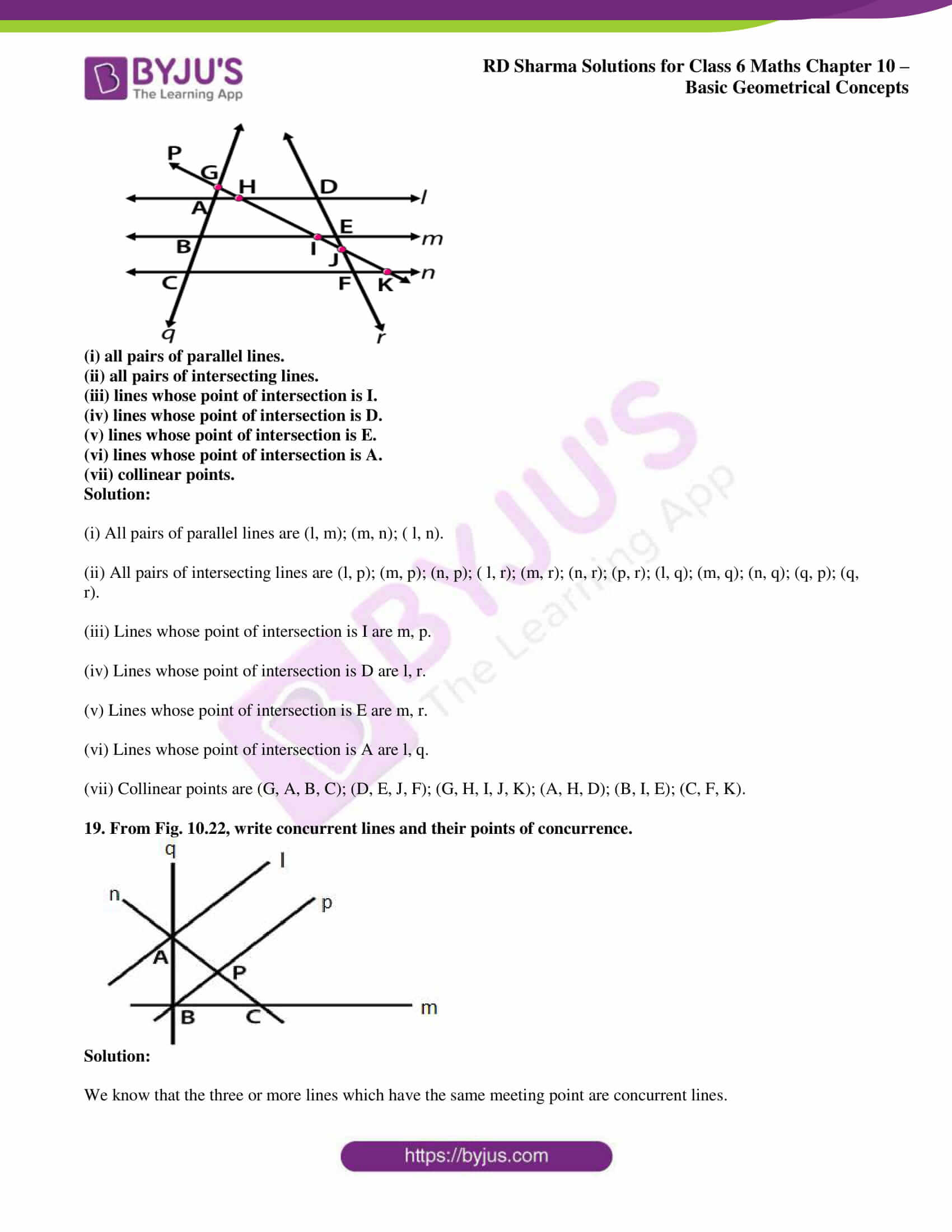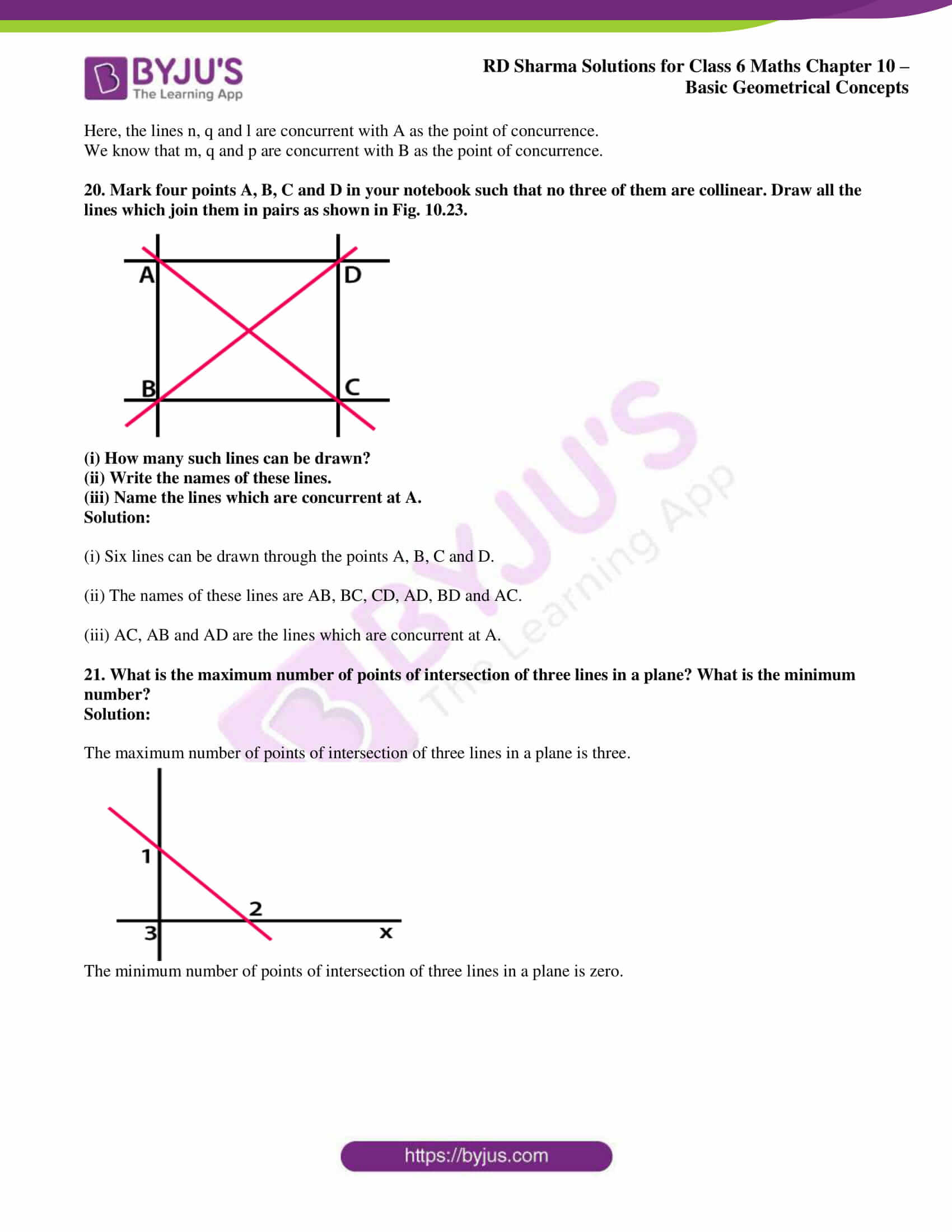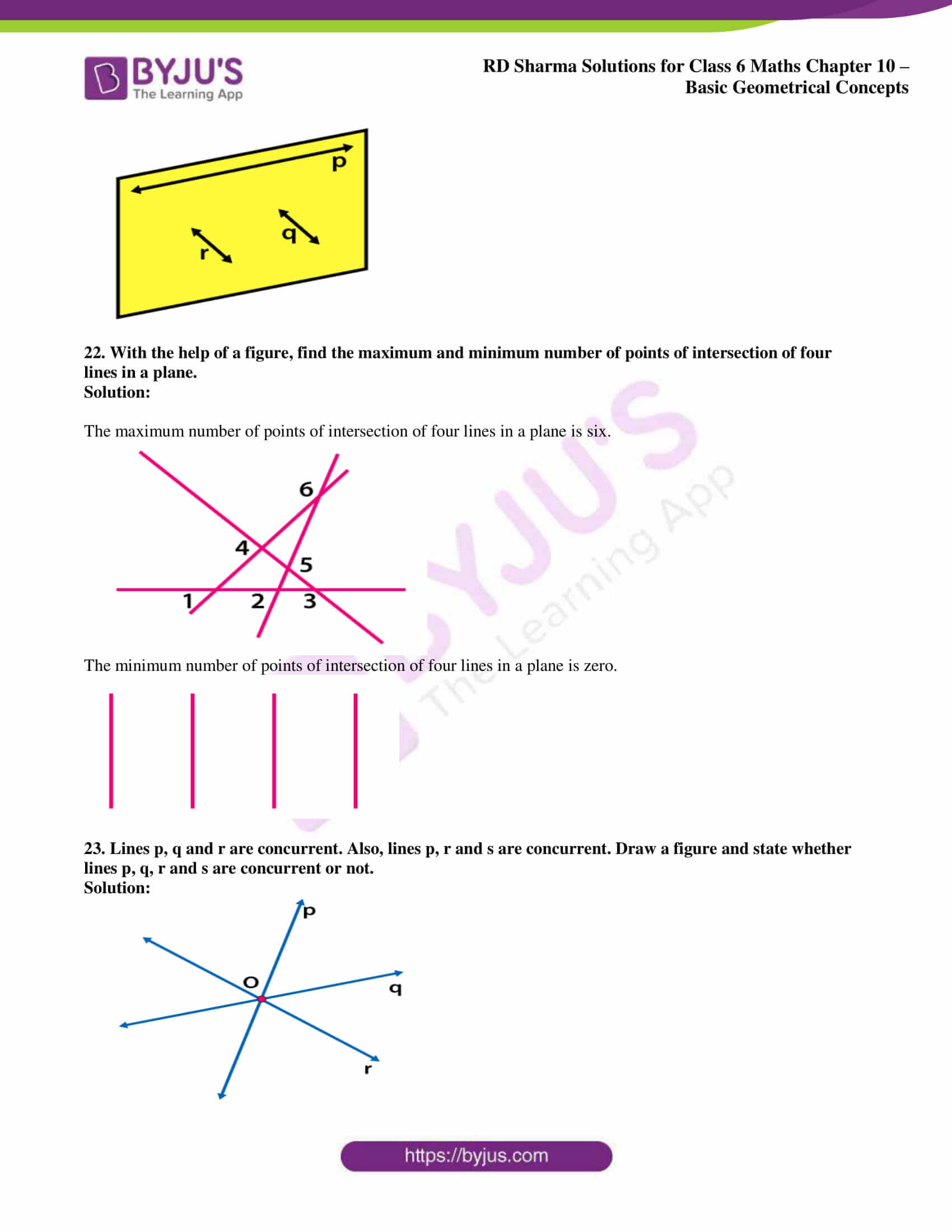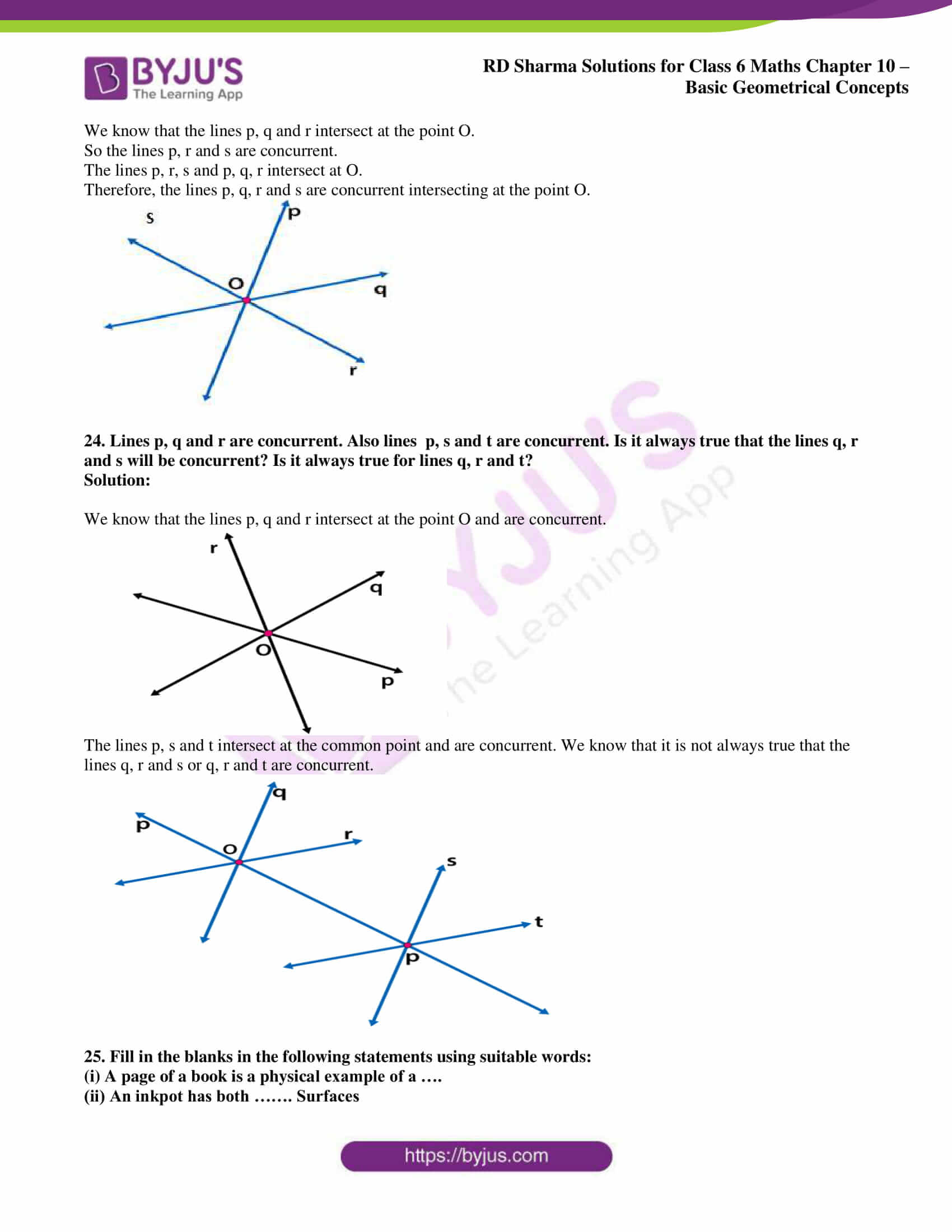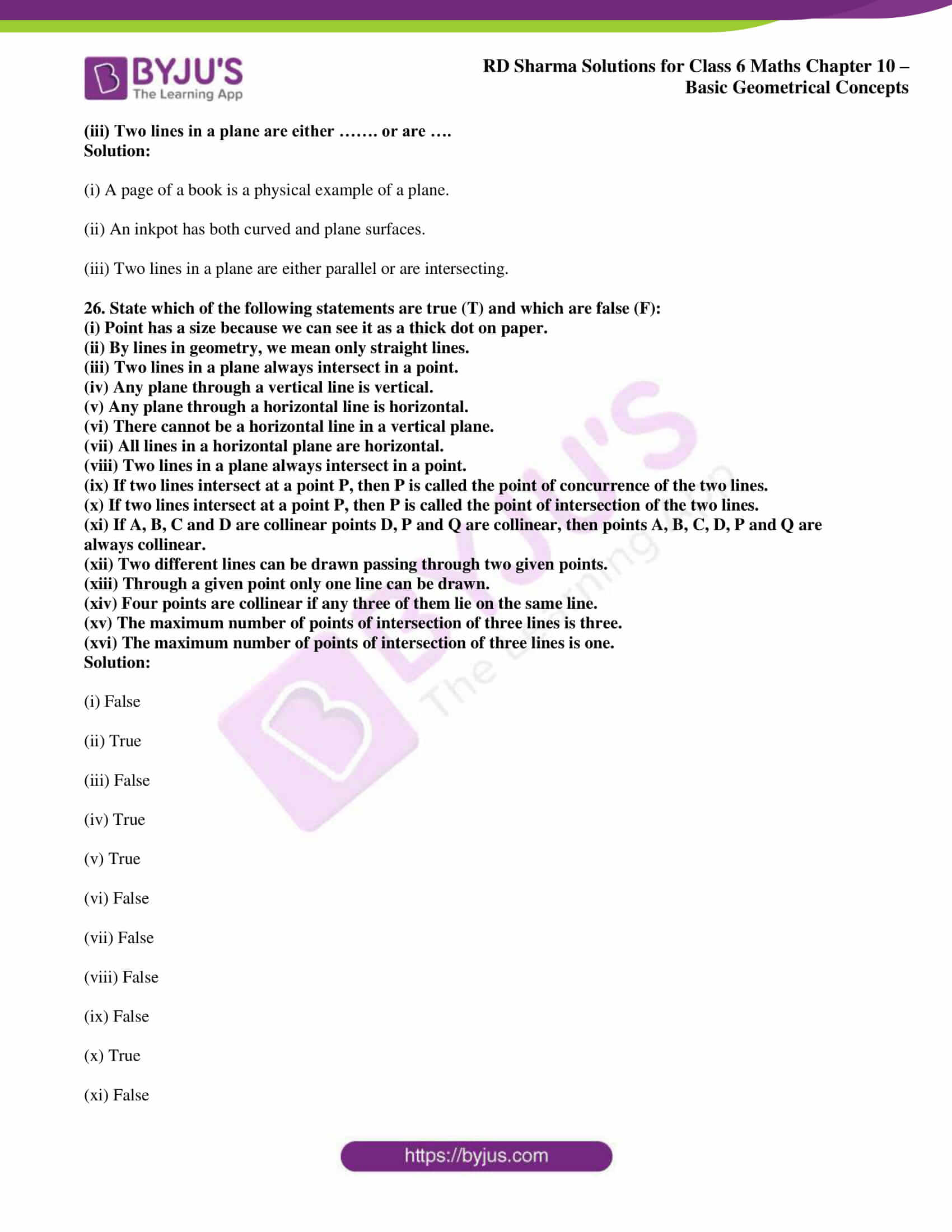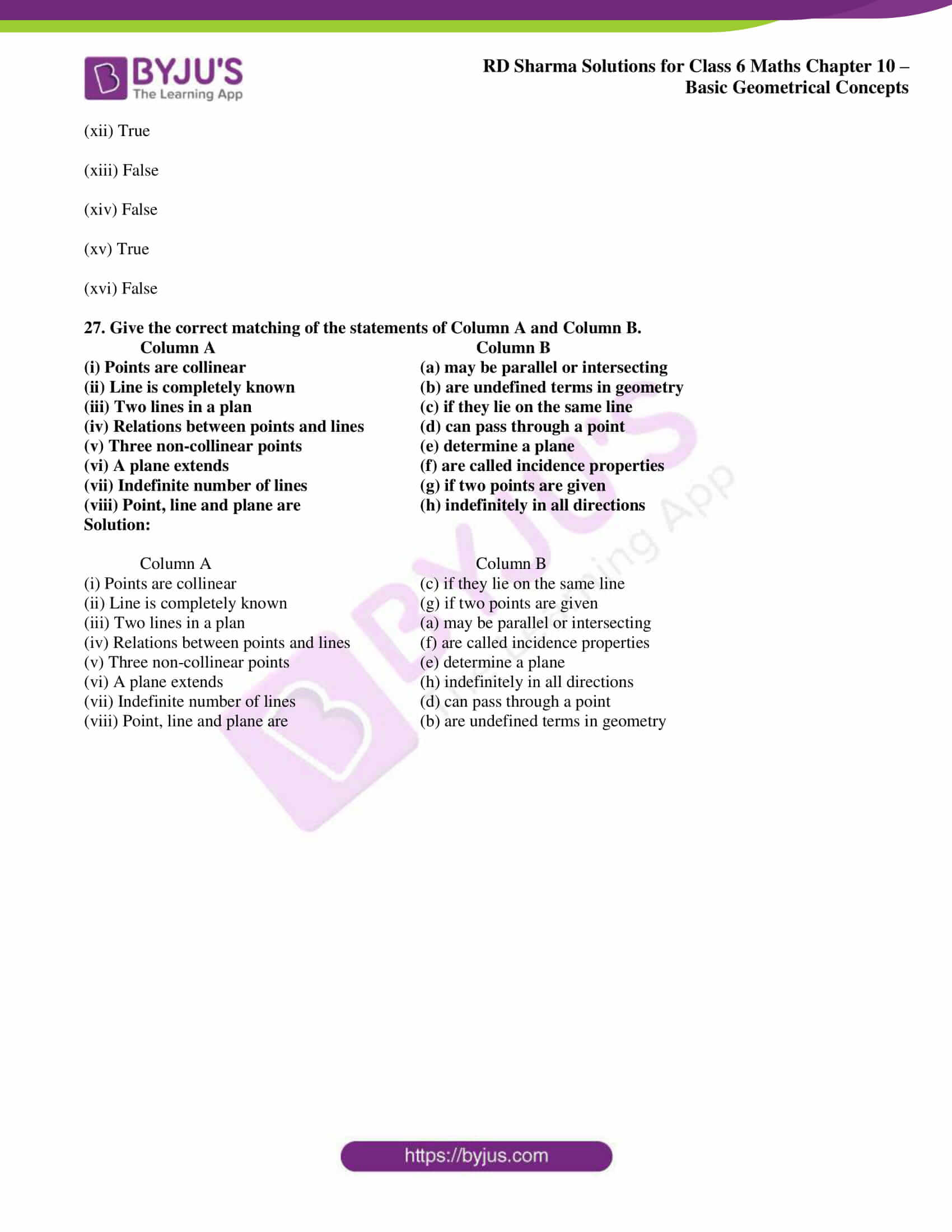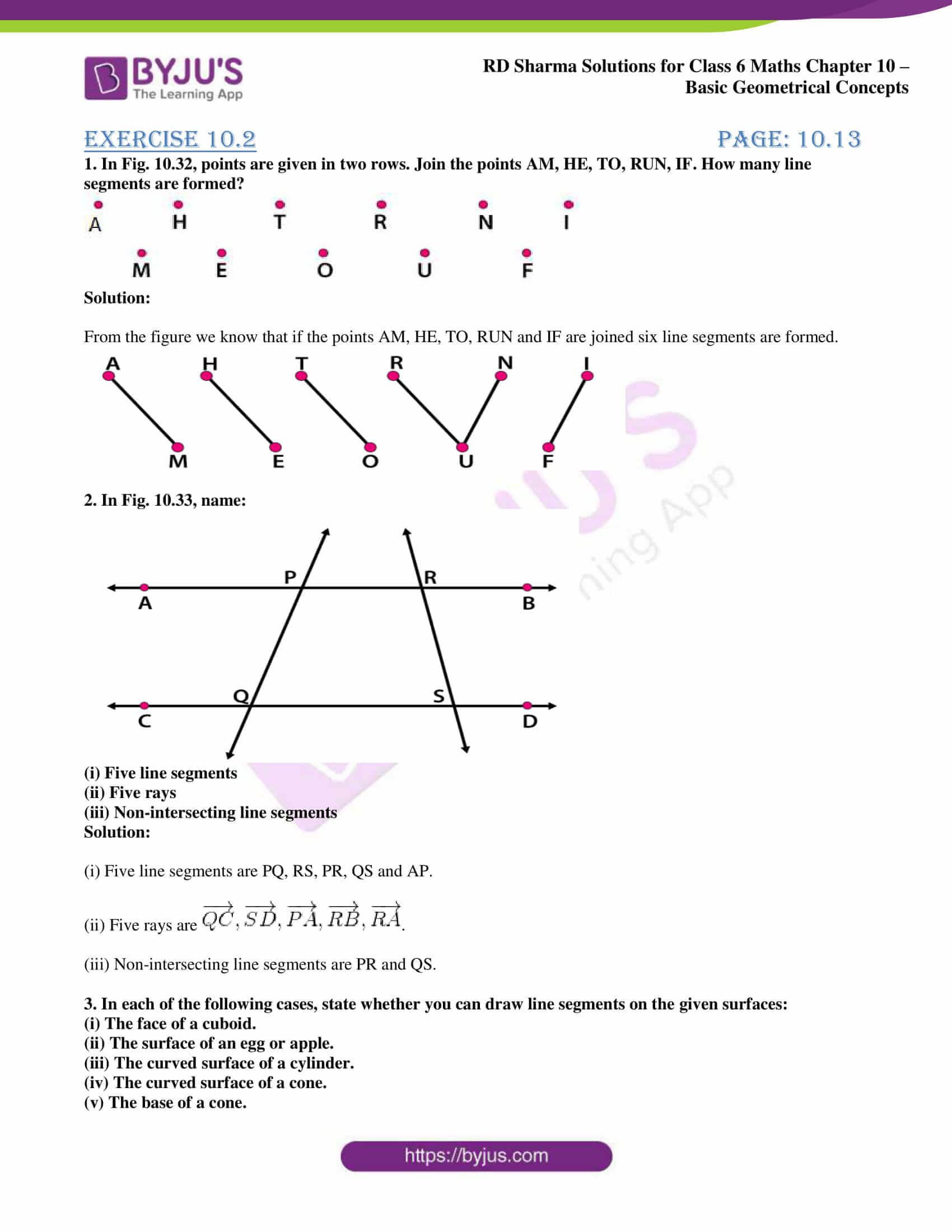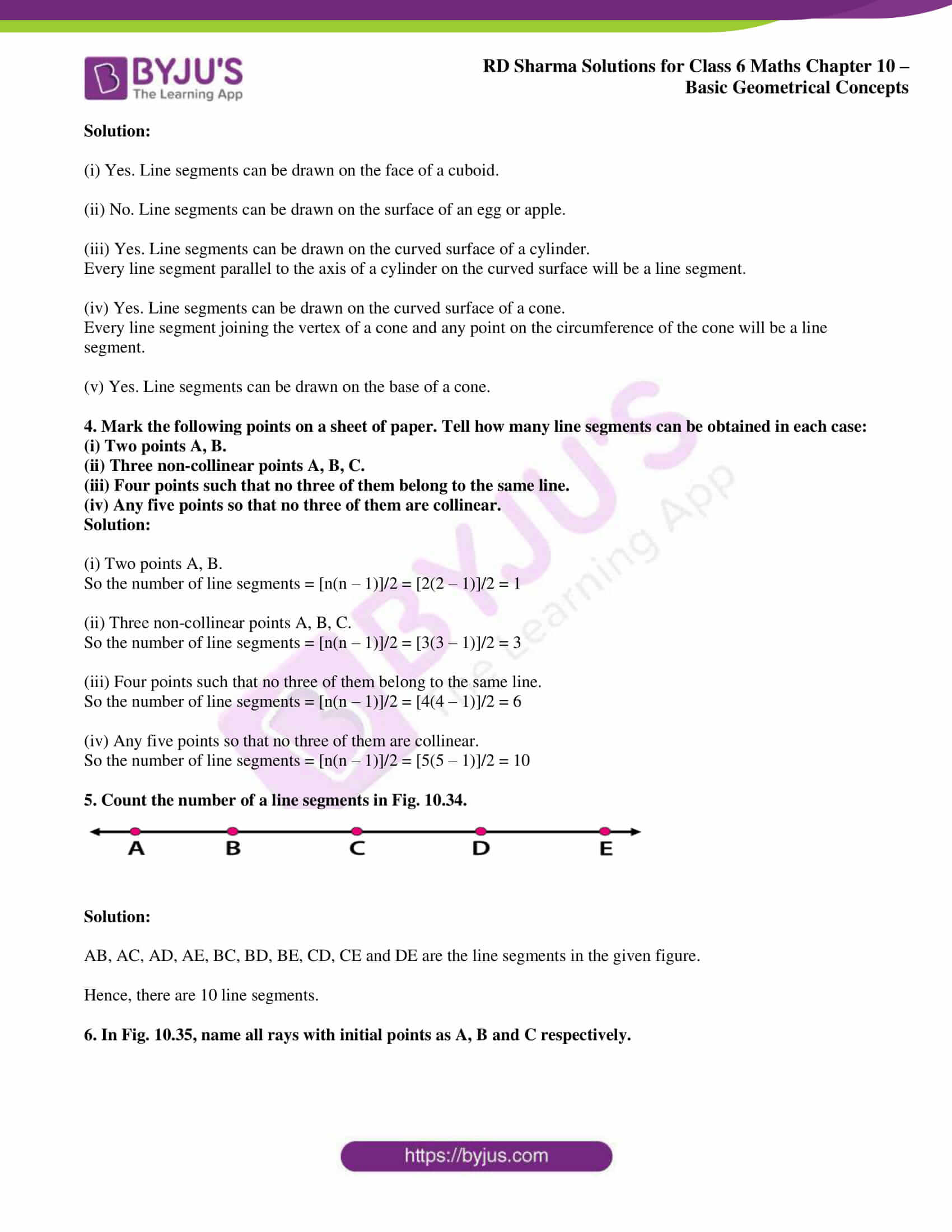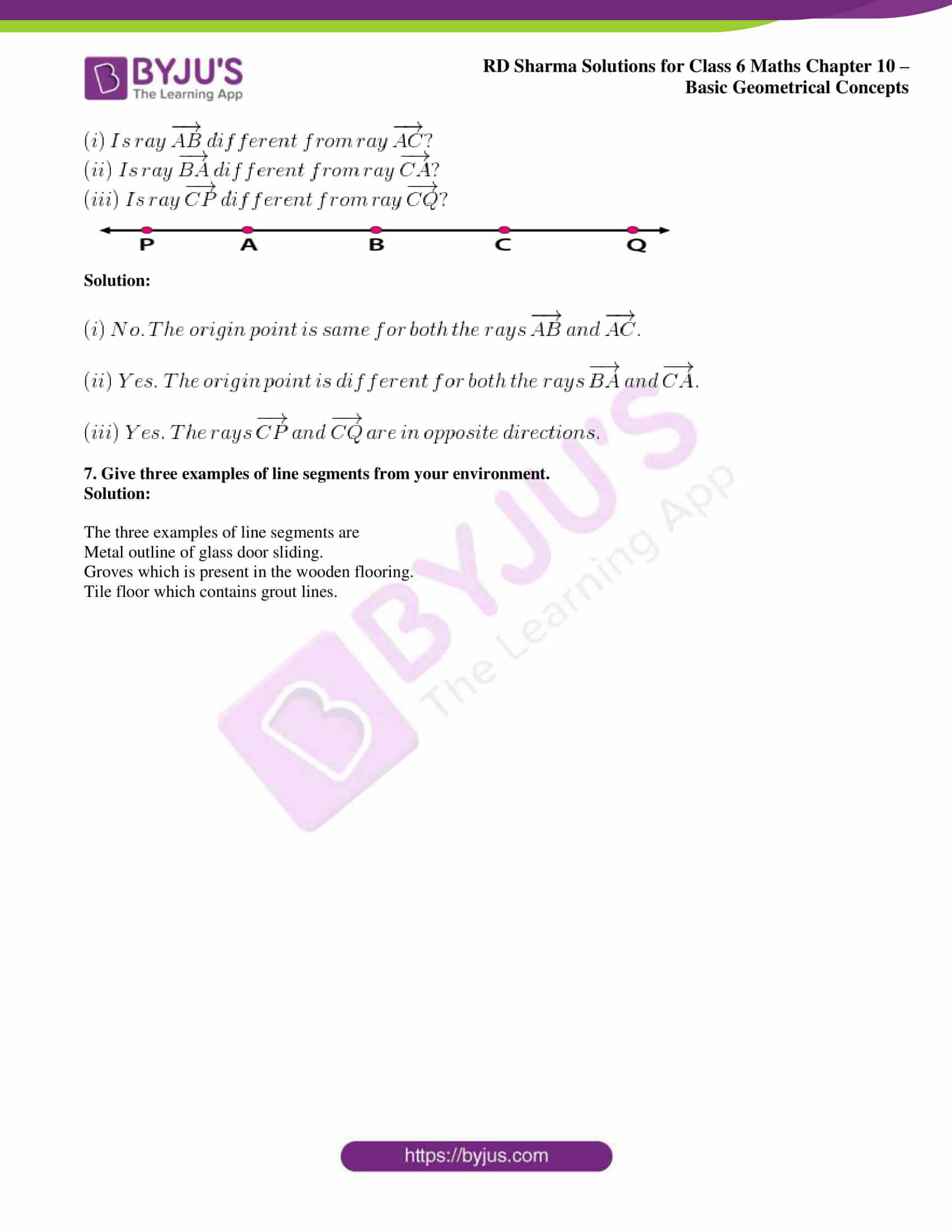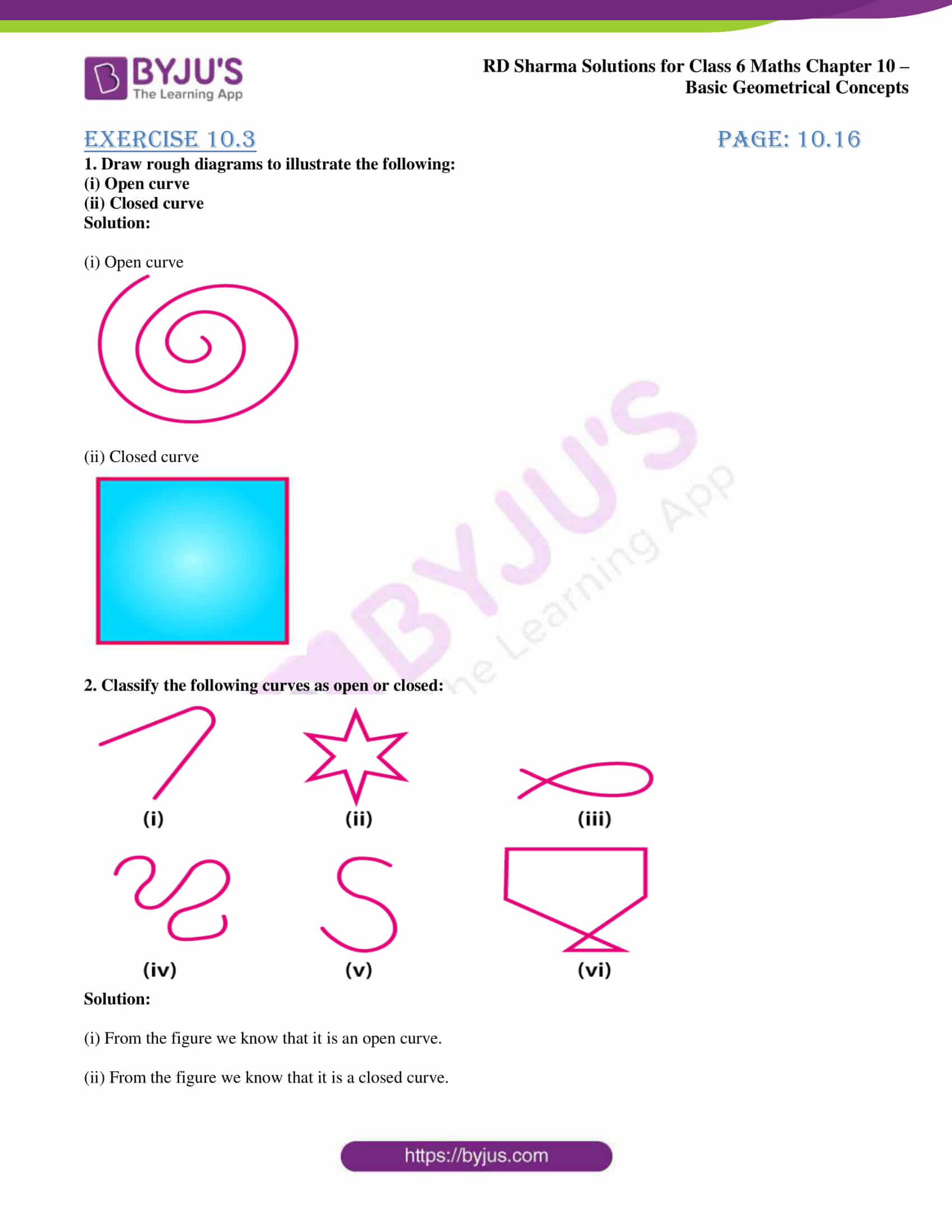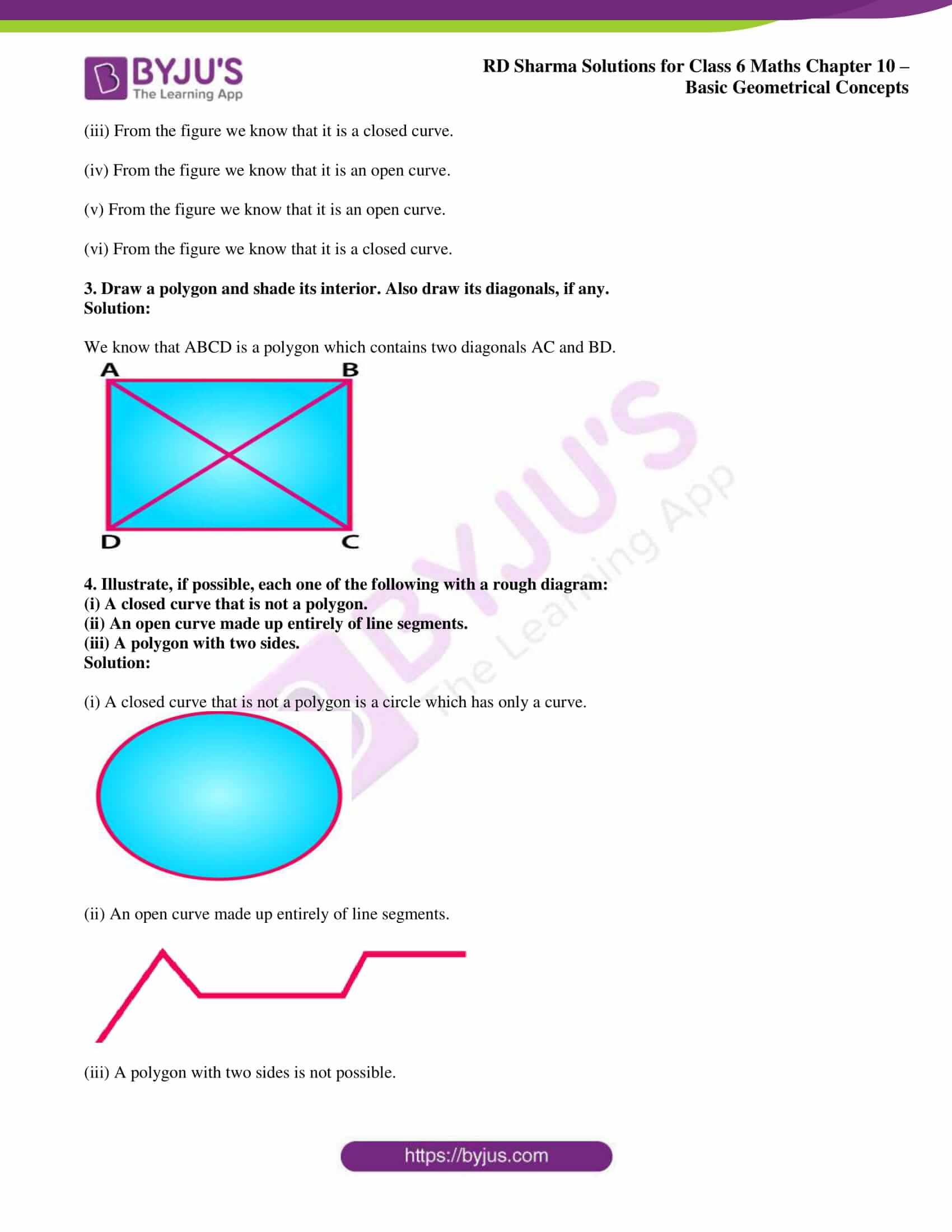## Access answers to Maths RD Sharma Solutions for Class 6 Chapter 10: Basic Geometrical Concepts

### Exercise 10.1 page: 10.7

1. Make three points in your notebook and name them.

Solution:

The three points A, P and H are marked as given below: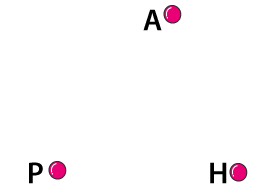2. Draw a line in your notebook and name it using a small letter of the alphabet.

Solution:

The line AB is drawn and is named as l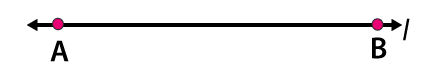3. Draw a line in your notebook and name it by taking any two points on it.

Solution:

Construct a line and name the points PQ. It can be called as the line PQ.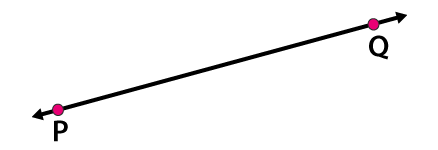4. Give three examples from your environment of:

(i) Points

(ii) Portion of a line

(iii) Plane surfaces

(iv) Portion of a plane

(v) Curved surfaces

Solution:

(i) Points

The three examples are

Pinhole on the map

Two walls and floor meeting at the corner

Period at the end of the sentence

(ii) Portion of a line

The three examples are

Thin curtain rods

Laser beams

Stretched power cables

(iii) Plane surfaces

The three examples are

Surface of a white board

Top of a table

Surface of a wall

(iv) Portion of a plane

The three examples are

Surface of a mirror

Calm water in a swimming pool

Surface of the sheet of paper

(v) Curved surfaces

The three examples are

Ink pot

Tea pot

Gas cylinder

5. There are a number of ways by which we can visualise a portion of a line. State whether the following represent a portion of a line or not:

(i) A piece of elastic stretched to the breaking point.

(ii) Wire between two electric poles.

(iii) The line thread by which a spider lowers itself.

Solution:

(i) Yes.

(ii) No.

(iii) Yes.

6. Can you draw a line on the surface of a sphere which lies wholly on it?

Solution:

No. A line cannot be drawn on the surface of the sphere which lies wholly on it.

7. Mark a point on a sheet of paper and draw a line passing through it. How many lines can you draw through this point?

Solution:

Unlimited number of lines can be drawn through the point L.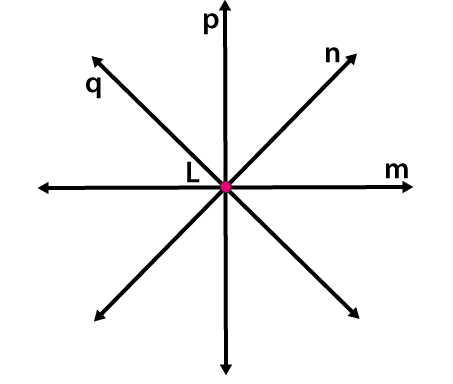8. Mark any two points P and Q in your note book and draw a line passing through the points. How many lines can you draw passing through both the points?

Solution:

Draw a line passing through the points P and Q

Only one line can be drawn passing through this both points.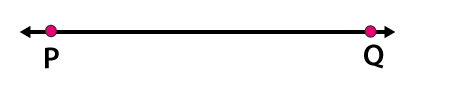9. Give an example of a horizontal plane and a vertical plane from your environment.

Solution:

The example of horizontal plane is ceiling of a room.

The example of a vertical plane is wall of a room.

10. How many lines may pass through one given point, two given points, any three collinear points?

Solution:

The lines passing through one given point is unlimited.The lines passing through two given points are only one.The lines passing through three collinear points are one.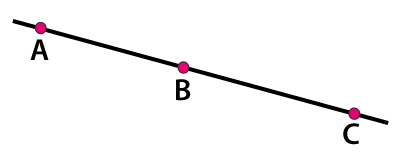11. Is it ever possible for exactly one line to pass through three points?

Solution:

Yes. It is possible for a line to pass through three points, if the points lie on a straight line.12. Explain why it is not possible for a line to have a mid-point.

Solution:

We know that the length of the line is infinite and it is not possible to find the midpoint.

But it is possible to find the midpoint of line segments.

13. Mark three non-collinear points A, B and C in your note book. Draw lines through these points taking two at a time. Name these lines. How many such different lines can be drawn?

Solution:

It is given that the three collinear points are A, B and C

We know that three lines namely AB, BC and AC can be drawn using these points.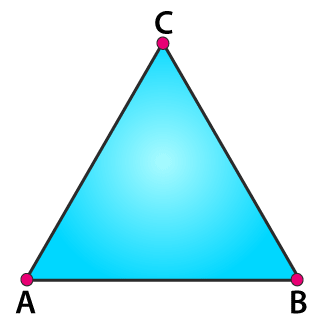14. Coplanar points are the points that are in the same plane. Thus,

(i) Can 150 points be coplanar?

(ii) Can 3 points be non-coplanar?

Solution:

(i) Yes. We know that the group of points which lie in the same plane are coplanar points.

Hence, 150 points can be coplanar.

(ii) No. We know that 3 points can be coplanar as we can have plane which contains 3 points.

Hence, 3 points cannot be non-coplanar.

15. Using a ruler, check whether the following points given in Fig. 10.30 are collinear or not:

(i) D, A and C

(ii) A, B and C

(iii) A, B and E

(iv) B, C and E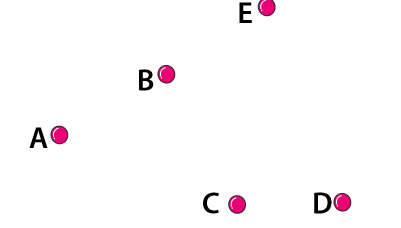Solution:

(i) The points D, A and C are collinear.

(ii) The points A, B and C are non-collinear.

(iii) The points A, B and E are collinear.

(iv) The points B, C and E are non-collinear.

16. Lines p, q are coplanar. So are the lines p, r. Can we conclude that the lines p, q, r are coplanar?

Solution:

Yes. The lines p, q and r are coplanar.

17. Give three examples each of:

(i) intersecting lines

(ii) parallel lines from your environment.

Solution:

(i) Three examples of intersecting lines are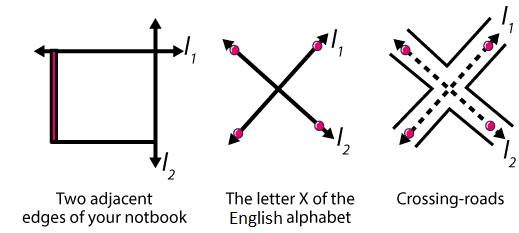(ii) Three examples of parallel lines from your environment are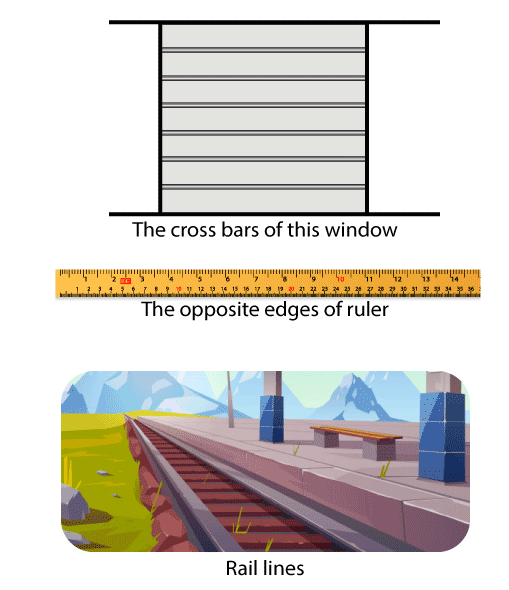18. From Fig. 10.21, write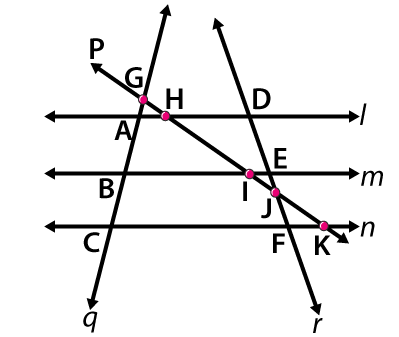(i) all pairs of parallel lines.

(ii) all pairs of intersecting lines.

(iii) lines whose point of intersection is I.

(iv) lines whose point of intersection is D.

(v) lines whose point of intersection is E.

(vi) lines whose point of intersection is A.

(vii) collinear points.

Solution:

(i) All pairs of parallel lines are (l, m); (m, n); ( l, n).

(ii) All pairs of intersecting lines are (l, p); (m, p); (n, p); ( l, r); (m, r); (n, r); (p, r); (l, q); (m, q); (n, q); (q, p); (q, r).

(iii) Lines whose point of intersection is I are m, p.

(iv) Lines whose point of intersection is D are l, r.

(v) Lines whose point of intersection is E are m, r.

(vi) Lines whose point of intersection is A are l, q.

(vii) Collinear points are (G, A, B, C); (D, E, J, F); (G, H, I, J, K); (A, H, D); (B, I, E); (C, F, K).

19. From Fig. 10.22, write concurrent lines and their points of concurrence.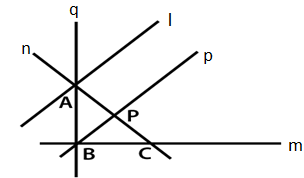Solution:

We know that the three or more lines which have the same meeting point are concurrent lines.

Here, the lines n, q and l are concurrent with A as the point of concurrence.

We know that m, q and p are concurrent with B as the point of concurrence.

20. Mark four points A, B, C and D in your notebook such that no three of them are collinear. Draw all the lines which join them in pairs as shown in Fig. 10.23.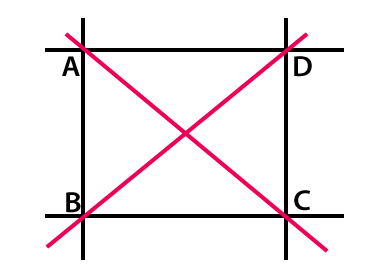(i) How many such lines can be drawn?

(ii) Write the names of these lines.

(iii) Name the lines which are concurrent at A.

Solution:

(i) Six lines can be drawn through the points A, B, C and D.

(ii) The names of these lines are AB, BC, CD, AD, BD and AC.

(iii) AC, AB and AD are the lines which are concurrent at A.

21. What is the maximum number of points of intersection of three lines in a plane? What is the minimum number?

Solution:

The maximum number of points of intersection of three lines in a plane is three.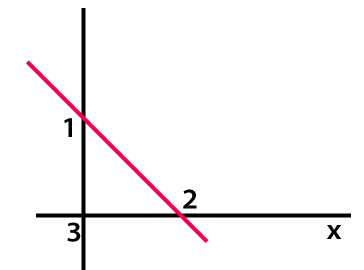The minimum number of points of intersection of three lines in a plane is zero.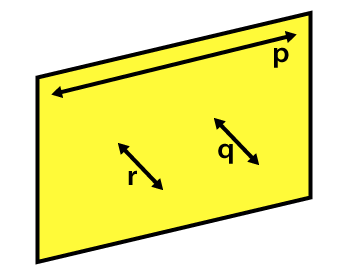22. With the help of a figure, find the maximum and minimum number of points of intersection of four lines in a plane.

Solution:

The maximum number of points of intersection of four lines in a plane is six.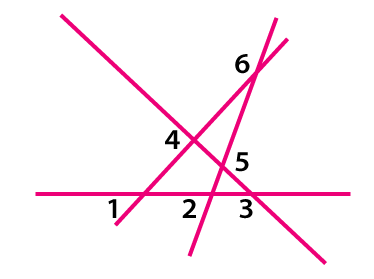The minimum number of points of intersection of four lines in a plane is zero.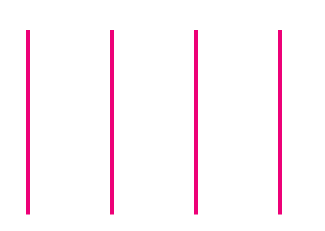23. Lines p, q and r are concurrent. Also, lines p, r and s are concurrent. Draw a figure and state whether lines p, q, r and s are concurrent or not.

Solution: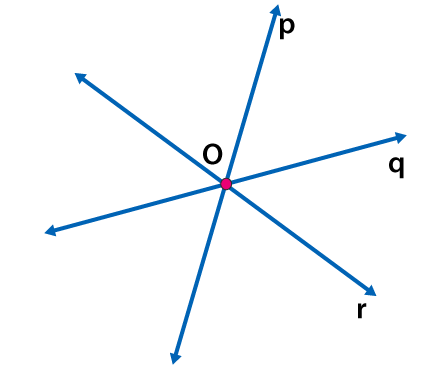We know that the lines p, q and r intersect at the point O.

So the lines p, r and s are concurrent.

The lines p, r, s and p, q, r intersect at O.

Therefore, the lines p, q, r and s are concurrent intersecting at the point O.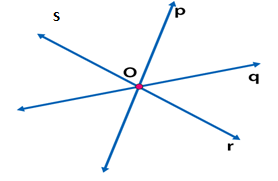24. Lines p, q and r are concurrent. Also lines p, s and t are concurrent. Is it always true that the lines q, r and s will be concurrent? Is it always true for lines q, r and t?

Solution:

We know that the lines p, q and r intersect at the point O and are concurrent.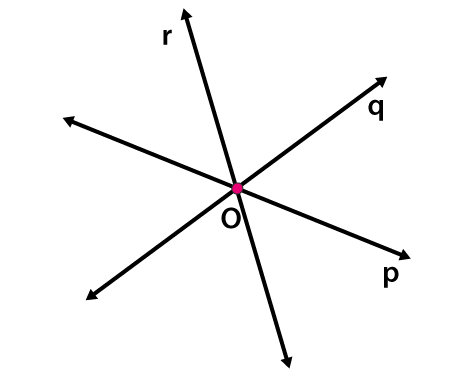The lines p, s and t intersect at the common point and are concurrent. We know that it is not always true that the lines q, r and s or q, r and t are concurrent.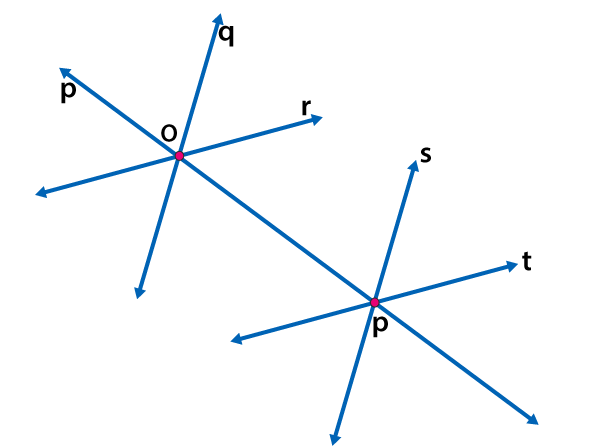25. Fill in the blanks in the following statements using suitable words:

(i) A page of a book is a physical example of a ….

(ii) An inkpot has both ……. Surfaces

(iii) Two lines in a plane are either ……. or are ….

Solution:

(i) A page of a book is a physical example of a plane.

(ii) An inkpot has both curved and plane surfaces.

(iii) Two lines in a plane are either parallel or are intersecting.

26. State which of the following statements are true (T) and which are false (F):

(i) Point has a size because we can see it as a thick dot on paper.

(ii) By lines in geometry, we mean only straight lines.

(iii) Two lines in a plane always intersect in a point.

(iv) Any plane through a vertical line is vertical.

(v) Any plane through a horizontal line is horizontal.

(vi) There cannot be a horizontal line in a vertical plane.

(vii) All lines in a horizontal plane are horizontal.

(viii) Two lines in a plane always intersect in a point.

(ix) If two lines intersect at a point P, then P is called the point of concurrence of the two lines.

(x) If two lines intersect at a point P, then P is called the point of intersection of the two lines.

(xi) If A, B, C and D are collinear points D, P and Q are collinear, then points A, B, C, D, P and Q are always collinear.

(xii) Two different lines can be drawn passing through two given points.

(xiii) Through a given point only one line can be drawn.

(xiv) Four points are collinear if any three of them lie on the same line.

(xv) The maximum number of points of intersection of three lines is three.

(xvi) The maximum number of points of intersection of three lines is one.

Solution:

(i) False

(ii) True

(iii) False

(iv) True

(v) True

(vi) False

(vii) False

(viii) False

(ix) False

(x) True

(xi) False

(xii) True

(xiii) False

(xiv) False

(xv) True

(xvi) False

27. Give the correct matching of the statements of Column A and Column B.

Column A Column B

(i) Points are collinear (a) may be parallel or intersecting

(ii) Line is completely known (b) are undefined terms in geometry

(iii) Two lines in a plan (c) if they lie on the same line

(iv) Relations between points and lines (d) can pass through a point

(v) Three non-collinear points (e) determine a plane

(vi) A plane extends (f) are called incidence properties

(vii) Indefinite number of lines (g) if two points are given

(viii) Point, line and plane are (h) indefinitely in all directions

Solution:

Column A Column B

(i) Points are collinear (c) if they lie on the same line

(ii) Line is completely known (g) if two points are given

(iii) Two lines in a plan (a) may be parallel or intersecting

(iv) Relations between points and lines (f) are called incidence properties

(v) Three non-collinear points (e) determine a plane

(vi) A plane extends (h) indefinitely in all directions

(vii) Indefinite number of lines (d) can pass through a point

(viii) Point, line and plane are (b) are undefined terms in geometry

### Exercise 10.2 page: 10.13

1. In Fig. 10.32, points are given in two rows. Join the points AM, HE, TO, RUN, IF. How many line segments are formed?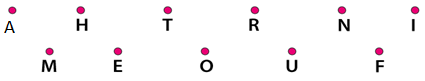Solution:

From the figure we know that if the points AM, HE, TO, RUN and IF are joined six line segments are formed.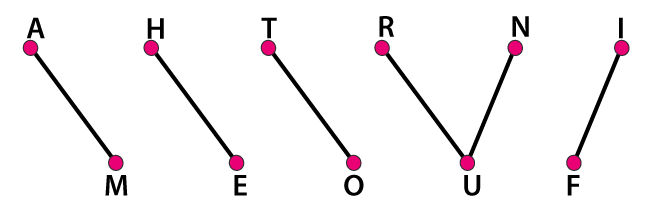2. In Fig. 10.33, name: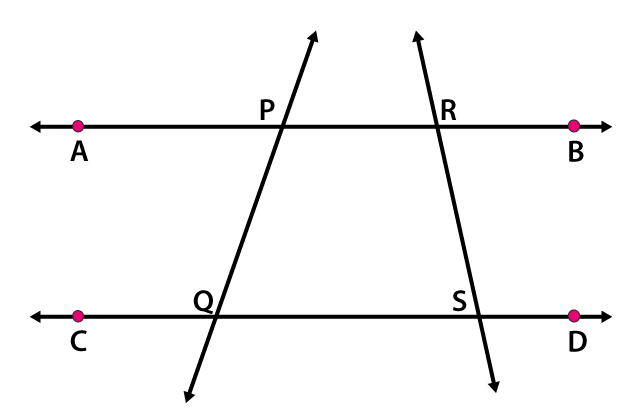(i) Five line segments

(ii) Five rays

(iii) Non-intersecting line segments

Solution:

(i) Five line segments are PQ, RS, PR, QS and AP.

(ii) Five rays are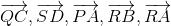.

(iii) Non-intersecting line segments are PR and QS.

3. In each of the following cases, state whether you can draw line segments on the given surfaces:

(i) The face of a cuboid.

(ii) The surface of an egg or apple.

(iii) The curved surface of a cylinder.

(iv) The curved surface of a cone.

(v) The base of a cone.

Solution:

(i) Yes. Line segments can be drawn on the face of a cuboid.

(ii) No. Line segments can be drawn on the surface of an egg or apple.

(iii) Yes. Line segments can be drawn on the curved surface of a cylinder.

Every line segment parallel to the axis of a cylinder on the curved surface will be a line segment.

(iv) Yes. Line segments can be drawn on the curved surface of a cone.

Every line segment joining the vertex of a cone and any point on the circumference of the cone will be a line segment.

(v) Yes. Line segments can be drawn on the base of a cone.

4. Mark the following points on a sheet of paper. Tell how many line segments can be obtained in each case:

(i) Two points A, B.

(ii) Three non-collinear points A, B, C.

(iii) Four points such that no three of them belong to the same line.

(iv) Any five points so that no three of them are collinear.

Solution:

(i) Two points A, B.

So the number of line segments = [n(n – 1)]/2 = [2(2 – 1)]/2 = 1

(ii) Three non-collinear points A, B, C.

So the number of line segments = [n(n – 1)]/2 = [3(3 – 1)]/2 = 3

(iii) Four points such that no three of them belong to the same line.

So the number of line segments = [n(n – 1)]/2 = [4(4 – 1)]/2 = 6

(iv) Any five points so that no three of them are collinear.

So the number of line segments = [n(n – 1)]/2 = [5(5 – 1)]/2 = 10

5. Count the number of a line segments in Fig. 10.34.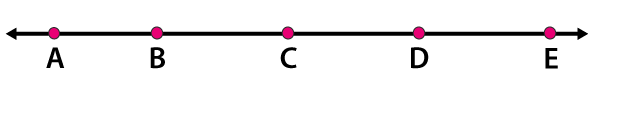Solution:

AB, AC, AD, AE, BC, BD, BE, CD, CE and DE are the line segments in the given figure.

Hence, there are 10 line segments.

6. In Fig. 10.35, name all rays with initial points as A, B and C respectively.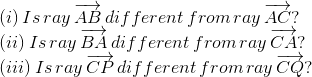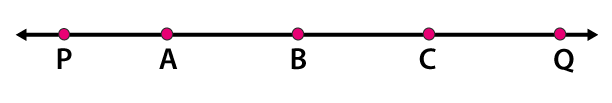Solution: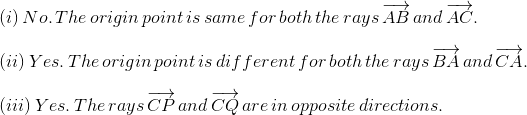7. Give three examples of line segments from your environment.

Solution:

The three examples of line segments are

Metal outline of glass door sliding.

Groves which is present in the wooden flooring.

Tile floor which contains grout lines.

### Exercise 10.3 page: 10.16

1. Draw rough diagrams to illustrate the following:

(i) Open curve

(ii) Closed curve

Solution:

(i) Open curve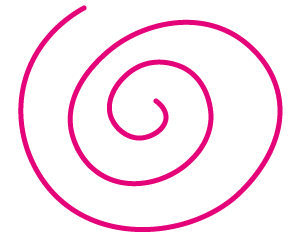(ii) Closed curve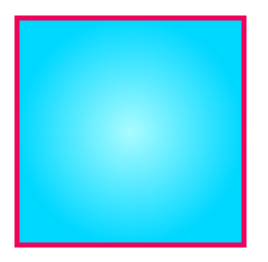2. Classify the following curves as open or closed: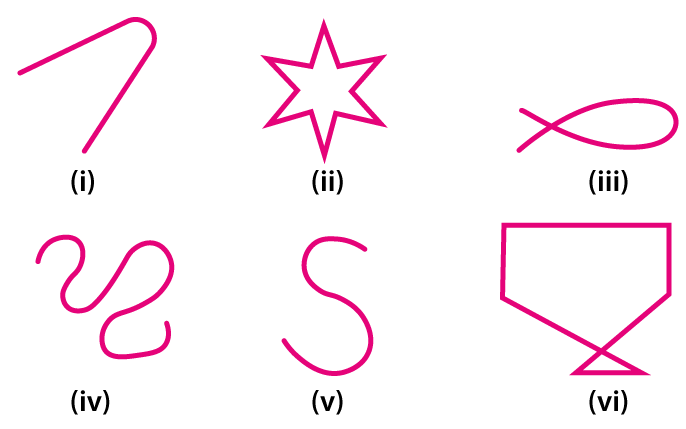Solution:

(i) From the figure we know that it is an open curve.

(ii) From the figure we know that it is a closed curve.

(iii) From the figure we know that it is a closed curve.

(iv) From the figure we know that it is an open curve.

(v) From the figure we know that it is an open curve.

(vi) From the figure we know that it is a closed curve.

3. Draw a polygon and shade its interior. Also draw its diagonals, if any.

Solution:

We know that ABCD is a polygon which contains two diagonals AC and BD.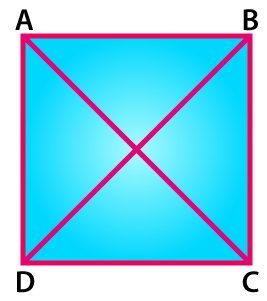4. Illustrate, if possible, each one of the following with a rough diagram:

(i) A closed curve that is not a polygon.

(ii) An open curve made up entirely of line segments.

(iii) A polygon with two sides.

Solution:

(i) A closed curve that is not a polygon is a circle which has only a curve.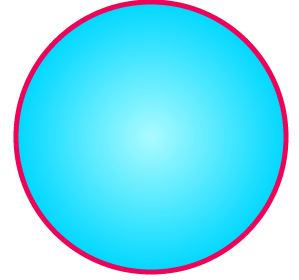(ii) An open curve made up entirely of line segments.(iii) A polygon with two sides is not possible.